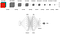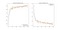# Galaxies Morphology Classification Using Convolutional Neural Networks

The Universe is made of clusters of millions of galaxies of different shapes and sizes. The study of galaxies and their evolution plays an important role in understanding how the Universe works. The classification of galaxies it’s useful while studying how galaxies are formed, and how they evolve. Current surveys of the sky produce huge amounts of data, manually labeling galaxies’ morphological features can be time consuming, and prone to errors, so people are trying to come up with clever ways to tag these automatically. The goal of this work is to build a model that can predict the type of galaxy given an image in the visible spectrum.

The galaxy-convnet GitHub repository includes notebooks for the creation of the dataset, and the training of the model with and without data augmentation, discussed in the next sections.

The goal is to be able to classify images in the visual spectrum. We are aiming at 3 types of classes only: spiral, elliptical and somewhere in-between (lenticular). The initial dataset is available from the Galaxy Zoo challenge that contains a total of 61578 images. Each image is a galaxy, and for each image volunteers answered a set of questions to help identify special characteristics of the galaxy (e.g. spiral or disk features).

In order to train our classification model we need a set of images for each of the three classes of interest. So we devise a path in the decision tree to group images of the same class. For example, we consider elliptical galaxies, the images where 80% of the people considered the galaxy smooth (opposed to having features/disk), and that at least 40% considered the galaxy completely round. A similar approach was devised for the other classes, the following figure illustrates some examples for each type of galaxy.

A Python notebook illustrating the creation of the dataset used is available in the galaxy-convnet GitHub repository.

To build a model to predict the galaxy type we used a CNN, due to it’s usually good results for classification images tasks. The following figure illustrates the sequence of convolutional, pooling and fully connected layers that are composed together to build our model.CNN to predict type of galaxy from a color 150x150 image.

We divided the dataset created in two sets: training and testing, using the test set to validate the model at each training epoch. The following plot shows the accuracy of the training and validation set when training the model for 100 epochs.

It seems to indicate that we are starting to have a bit of an over-fitting, we are approaching 100% accuracy in the training accuracy, while the accuracy in the validation set has stagnated around 85%. To try to overcame this problem we used a technique usually refereed to as augmented data, i.e. we generate batches of images by randomly applying some image transformation increasing the variance of the training set for each training epoch. The same plots as before are illustrated in the following figure but this time training the model using data augmentation.Model training and validation accuracy and loss function plots using augmented data.

From a visual analysis of the plots we see that when using augmented data the validation accuracy climbs steady with the training accuracy, giving an hint that the model it not over-fitting to the training data.

# Testing the Model

Finally we do some testing of the model with some random images found online. Given an image the model outputs a list of 3 values, where each value is the probability of the galaxy to belong to the elliptical class, the lenticular class, or the spiral class respectively. The following images illustrate the model ability to predict the morphological class of images not seen in the training/test sets.